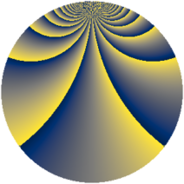# Properties

 Label 2280.2.egLevel $2280$ Weight $2$ Character orbit 2280.eg Rep. character $\chi_{2280}(899,\cdot)$ Character field $\Q(\zeta_{18})$ Dimension $2832$ Sturm bound $960$

# Related objects

## Defining parameters

 Level: $$N$$ $$=$$ $$2280 = 2^{3} \cdot 3 \cdot 5 \cdot 19$$ Weight: $$k$$ $$=$$ $$2$$ Character orbit: $$[\chi]$$ $$=$$ 2280.eg (of order $$18$$ and degree $$6$$) Character conductor: $$\operatorname{cond}(\chi)$$ $$=$$ $$2280$$ Character field: $$\Q(\zeta_{18})$$ Sturm bound: $$960$$

## Dimensions

The following table gives the dimensions of various subspaces of $$M_{2}(2280, [\chi])$$.

Total New Old
Modular forms 2928 2928 0
Cusp forms 2832 2832 0
Eisenstein series 96 96 0

## Trace form

 $$2832q - 30q^{4} - 12q^{6} - 24q^{9} + O(q^{10})$$ $$2832q - 30q^{4} - 12q^{6} - 24q^{9} - 12q^{10} - 18q^{16} - 48q^{19} + 48q^{24} - 24q^{25} + 18q^{30} + 18q^{34} - 12q^{36} - 42q^{40} - 12q^{46} - 1296q^{49} - 24q^{51} - 60q^{60} + 30q^{64} - 48q^{66} + 66q^{70} - 192q^{75} - 60q^{76} - 24q^{81} + 78q^{84} + 18q^{90} + 120q^{91} - 24q^{94} + 24q^{96} - 132q^{99} + O(q^{100})$$

## Decomposition of $$S_{2}^{\mathrm{new}}(2280, [\chi])$$ into newform subspaces

The newforms in this space have not yet been added to the LMFDB.